SSC  >  Quantitative Aptitude - Test 6

# Quantitative Aptitude - Test 6

Test Description

## 25 Questions MCQ Test SSC CGL Tier 1 Mock Test Series | Quantitative Aptitude - Test 6

Quantitative Aptitude - Test 6 for SSC 2022 is part of SSC CGL Tier 1 Mock Test Series preparation. The Quantitative Aptitude - Test 6 questions and answers have been prepared according to the SSC exam syllabus.The Quantitative Aptitude - Test 6 MCQs are made for SSC 2022 Exam. Find important definitions, questions, notes, meanings, examples, exercises, MCQs and online tests for Quantitative Aptitude - Test 6 below.
Solutions of Quantitative Aptitude - Test 6 questions in English are available as part of our SSC CGL Tier 1 Mock Test Series for SSC & Quantitative Aptitude - Test 6 solutions in Hindi for SSC CGL Tier 1 Mock Test Series course. Download more important topics, notes, lectures and mock test series for SSC Exam by signing up for free. Attempt Quantitative Aptitude - Test 6 | 25 questions in 15 minutes | Mock test for SSC preparation | Free important questions MCQ to study SSC CGL Tier 1 Mock Test Series for SSC Exam | Download free PDF with solutions
 1 Crore+ students have signed up on EduRev. Have you?
Quantitative Aptitude - Test 6 - Question 1

### Directions: The pie chart given below represents the modes of transport for 1400 employees of the staff Selection Commission, Kolkata. Study the chart and answer the questions.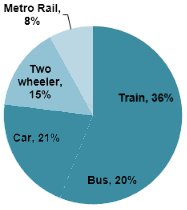Question: The number of employees who go to office by Metro Rail is

Detailed Solution for Quantitative Aptitude - Test 6 - Question 1

From the pie chart 8% of total employees go to office by metro rail.
Total employees = 1400
∴ Number of employees going to office by Metro rail = 8% of 1400 = 112
∴ 112 employees go to office by metro rail.

Quantitative Aptitude - Test 6 - Question 2

### Directions: The pie chart given below represents the modes of transport for 1400 employees of the staff Selection Commission, Kolkata. Study the chart and answer the questions.Question: The number of employees who go to office by car is

Detailed Solution for Quantitative Aptitude - Test 6 - Question 2

From the pie chart 21% of total employees go to office by car.
Total employees = 1400
Number of employees going to office by car = 21% of 1400 = 294
∴ 294 employees go to office by car.

Quantitative Aptitude - Test 6 - Question 3

### Directions: The pie chart given below represents the modes of transport for 1400 employees of the staff Selection Commission, Kolkata. Study the chart and answer the questions.Question: The ratio of two wheelers and cars being used as modes of transport is

Detailed Solution for Quantitative Aptitude - Test 6 - Question 3

From above question 294 employees go to office by car.
From the pie chart 15% of total employees go to office by two wheelers.
Total employees = 1400
Number of employees going to office by two wheelers = 15% of 1400 = 210
∴ 210 employees go to office by two wheelers.
∴ The ratio of two wheelers and cars being used as modes of transport is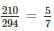Quantitative Aptitude - Test 6 - Question 4

Directions: The pie chart given below represents the modes of transport for 1400 employees of the staff Selection Commission, Kolkata. Study the chart and answer the questions.Question: Write down the difference: (Employees availing train - employees availing car)

Detailed Solution for Quantitative Aptitude - Test 6 - Question 4

From above question 294 employees go to office by car.
From the pie chart 36% of total employees go to office by train.
Total employees = 1400
Number of employees going to office by train = 36% of 1400 = 504
∴ 504 employees go to office by train.
∴ Employees availing train - employees availing car = (504 – 294) = 210

Quantitative Aptitude - Test 6 - Question 5

O is the center of circle and ∠QPS = 65°. Find the value of ∠A and ∠B respectively.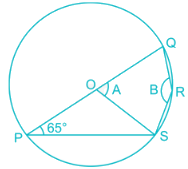Detailed Solution for Quantitative Aptitude - Test 6 - Question 5

We know that angle subtended by any two points from the circumference of the circle on the center is twice the angle subtended by those two points on any other part of the circle, so,
Points Q and S subtend an angle of 65° on the circumference so they subtend 65° × 2 = 130°.
Now, ∠ SOQ(outer) = 360° - 130° = 230°
Now similar to the previous part, ∠ SOQ(outer) is twice of angle B, so ∠ B = 230°/2 = 115°

Quantitative Aptitude - Test 6 - Question 6

Find the third vertex of a triangle, if two of its vertices are at (2, 3) and (4, -2) and the centroid is at (1, 1).

Detailed Solution for Quantitative Aptitude - Test 6 - Question 6

Centroid of a triangle = [(x1 + x2 + x3)/3 , (y1 + y2 + y3)/3] where (x, y1), (x2, y2) and (x3, y3) are co-ordinates of vertices of triangle
Given, two of its vertices are at (2, 3) and (4, -2) and the centroid is at (1, 1)
Let the coordinate of third vertices be (x, y)
∴ 1 = (2 + 4 + x)/3
⇒ x = - 3
And,
1 = (3 - 2 + y)/3
⇒ y = 2
So third coordinate is (-3, 2)

Quantitative Aptitude - Test 6 - Question 7

The side AC of the ΔABC is produced to D, such that CD = CB. If ∠ACB = 70°, then the value of ∠ADB is

Detailed Solution for Quantitative Aptitude - Test 6 - Question 7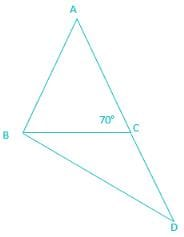Now we see that Δ ADB is isosceles as CD = CB and the sum of ∠ CBD and ∠ CDB is 70° (exterior angle property of a triangle). So,
∠CBD = ∠CDB    (isosceles triangle)
∠CBD + ∠CDB = 70°
⇒ 2 × ∠CDB = 70°
⇒ ∠ CDB = 35°

Quantitative Aptitude - Test 6 - Question 8

If x + (1/x) = 6, then find value of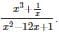Detailed Solution for Quantitative Aptitude - Test 6 - Question 8

We have,
x + (1/x) = 6
on squaring both side
⇒x+ 1/x2 = 36 – 2 = 34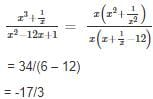Quantitative Aptitude - Test 6 - Question 9

The angles of depression of the top and bottom of a 50 m high electric pole form the top of a building are 30° and 60° respectively. Find the height of the building.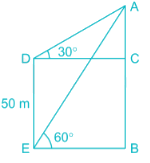Detailed Solution for Quantitative Aptitude - Test 6 - Question 9According to the figure,
DE is the electric pole (height = 50m), AB is the building.
∠ADC = 30° & ∠AEB = 60°
We have to find out the length of AB.
As, DC ⊥ AB & EB ⊥ AB so, DC || EB
⇒ DE = BC
Also, DE || AB so, DC = EB
Now, AD × cos30° = DC & AE × cos 60° = BE
⇒ AD × cos30° = AE × cos 60°
⇒ √3 ×AD = AE
From the figure, AB = AC + BC
⇒ AB = AC + DE [As, DE = BC]
⇒ AE × sin 60° = AD × sin 30° + 50
⇒ √3 ×AD × sin 60° = AD × sin 30° + 50
⇒ AD = 50
Now, AB = AD × sin 30° + 50
⇒ AB = 25 + 50 = 75 meter.
The height of the building = 75 meter

Quantitative Aptitude - Test 6 - Question 10

If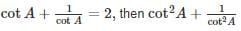is equal to

Detailed Solution for Quantitative Aptitude - Test 6 - Question 10

Formula:
(a + b)2 = a2 + b2 + 2ab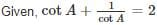Squaring both sides we get,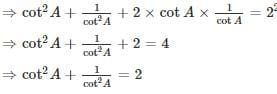Quantitative Aptitude - Test 6 - Question 11

The simplest value of cot9° cot27° cot63° cot 81° is

Detailed Solution for Quantitative Aptitude - Test 6 - Question 11

We know that cotθ = tan(90° - θ)
Cot63° = tan(90° - 63°)
Cot63° = tan27°
And cot81° = tan9°
cot9° cot27° cot63° cot 81°
= cot9° cot27° tan27° tan9°
We know that tanθcotθ = 1
= 1

Quantitative Aptitude - Test 6 - Question 12

If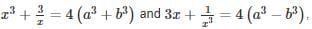then a2 – bis equal to

Detailed Solution for Quantitative Aptitude - Test 6 - Question 12

Formulas :-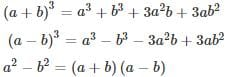Given the equation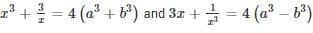Adding both the equation: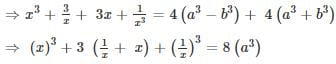Since we know a+ b3 + 3ab (a + b) = (a + b)3
⇒ (x + 1/x)3 = (2a)3
⇒ x + 1/x = 2a
Similarly, subtracting both the equation: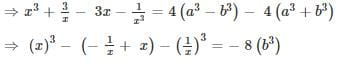Since we know a- b3 - 3ab (a - b) = (a - b)3
⇒ (x - 1/x)3 = (-2b)3
⇒ x - 1/x = -2b
Adding both the resultant equation,
⇒ x + 1/x + x – 1/x = 2a – 2b
⇒ 2x = 2a – 2b
⇒ x = a – b
Similarly, subtracting both the resultant equation,
⇒ x + 1/x - x + 1/x = 2a + 2b
⇒ 2/x = 2a + 2b
⇒ 1/x = a + b
Multiplying both the final equation:
⇒ (a + b)(a - b) = x × 1/x = 1
⇒ a2 – b2 = 1

Quantitative Aptitude - Test 6 - Question 13

ABC is an equilateral triangle with side 2 cm. With A, B and C as centres and radius 1 cm three arcs are drawn. The area of the region with in the triangle bounded by three arcs is

Detailed Solution for Quantitative Aptitude - Test 6 - Question 13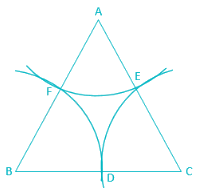ABC is an equilateral triangle
Given,
AB = BC = CA = 2 cm
∠A = ∠B = ∠C = 60°
Height of equilateral triangle = (√3/2) × (side)
= (√3/2) × 2 = √3 cm
∴ Area of the triangle = (1/2) × √3 × 2 = √3 cm2
Area inside the triangle inscribed by three arcs = 3 × area of one arc
= 3 × (θ/360°) × πr2
= 3 × π × 12 × (60/360) = π/2
∴ Required area = (√3 - π/2) cm2

Quantitative Aptitude - Test 6 - Question 14

ABCD is a parallelogram in which diagonals AC and BD intersect at O. If E, F, G and H are the mid points of AO, DO, CO and BO respectively, then the ratio of the perimeter of the quadrilateral EFGH to the perimeter of parallelogram ABCD is

Detailed Solution for Quantitative Aptitude - Test 6 - Question 14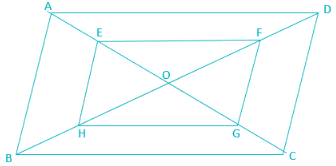In Δ OAB,
Mid- point of OA = E
Mid- point of OB = H
Therefore,
EH|| AB
Therefore,
HE = AB/2
Similarly,
HG = BC/2
FG = CD/2
EF + HG + FG + EF = (1/2)(AB + BC + CD + AD)
⇒ Perimeter of EFGH = (1/2) perimeter of ABCD
So,
The ratio of the perimeter of the quadrilateral EFGH to the perimeter of parallelogram ABCD = 1:2

Quantitative Aptitude - Test 6 - Question 15

If the roots of the equation ax2 + bx + c = 0 differ by 7, then which of the following expression shows the relation among a, b and c?

Detailed Solution for Quantitative Aptitude - Test 6 - Question 15

Roots of equation ax2 + bx + c = 0 are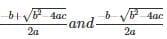Difference between the roots =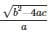Given, roots differ by 7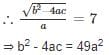Quantitative Aptitude - Test 6 - Question 16

A can do a piece of work in 18 days, B in 36 days and C in 72 days. All begin to do it together but A leaves after 8 days and B leaves 7 days before the completion of the work. The total number of days they worked for is

Detailed Solution for Quantitative Aptitude - Test 6 - Question 16

Given,
A can do a piece of work in 18 days
∴ Part of work done by A in 1 day = 1/18
B can do a piece of work in 36days
∴ Part of work done by B in 1 day = 1/36
C can do a piece of work in 72 days
∴ Part of work done by C in 1 day = 1/72
Let,
Total number of days they work = x days
Number of days A work = 8 days
∵ B leaves7 days before the completion of the work
Number of days B work = (x – 7) days
And, Number of days C work= x days
∴ {(1/18) × 8 } + {(1/36) × (x – 7)} + {(1/72) × x} = 1
⇒ 4/9 + (x – 7)/36 + x/72 = 1
⇒ (32 + 2x -14 + x)/72 = 1
⇒ 18 + 3x = 72
⇒ 3x = 54
⇒ x = 18 days

Quantitative Aptitude - Test 6 - Question 17

Form two places, 60 km apart, A and B start towards each other and meet after 6 hours. Had A travelled with 2/3 of his speed and B travelled with double of his speed, they would have met after 5 hours. The speed of A is:

Detailed Solution for Quantitative Aptitude - Test 6 - Question 17

Let speed of A be a kmph and speed of B be b kmph
Then we have
⇒ a + b = 60/6
⇒ a + b = 10
or 2a + 2b = 20------
also,
⇒ 2/3a + 2b = 60/5 = 12--------------
 –  leads to
⇒ (4/3)a = 8
a = 6 kmph

Quantitative Aptitude - Test 6 - Question 18

A container contains mixture of milk and water in the ratio of 4 : 7. 8 litres of this mixture is replaced by water. The new ratio of milk and water becomes 4 : 9. Find the initial quantity of water in the mixture.

Detailed Solution for Quantitative Aptitude - Test 6 - Question 18

Given, container contains mixture of milk and water in the ratio of 4 : 7.
Let the volume of milk and water be 4a and 7a.
Now, 8 litres are removed and replaced by water.
In 1 litre of mixture has 4/11 litres of milk and 7/11 litres of water are present.
Thus in 8 litres 32/11 and 56/11 litres of milk and water are present.
∴ Volume of milk = 4a – 32/11
Volume of water = 7a – 56/11 + 8
New ratio = 4 : 9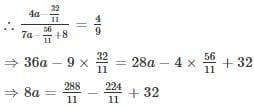⇒ a = 52/11
Now, initial amount of water = 7a = 364/11

Quantitative Aptitude - Test 6 - Question 19

Ram and Shyam start a joint business. Ram invests 1/3 of the total sum for 9 month and Shyam gets 2/5 of the profits. For how long, the investment of Shyam was in the business?

Detailed Solution for Quantitative Aptitude - Test 6 - Question 19

We know that investment × time = profit
Assume that the total sum = P & total profit is M
∴ Sum invested by Ram = ⅓P & sum invested by Shyam = ⅔P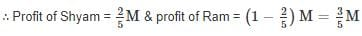For Ram we can say,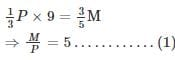For Shyam we can say,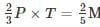[Here T = time for which investment of Shyam was in the business]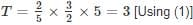∴ Investment of Shyam was in the business for 3 months.

Quantitative Aptitude - Test 6 - Question 20

The mean of 20 items is 55. If two items 45 and 30 are removed, the new mean of the remaining items is

Detailed Solution for Quantitative Aptitude - Test 6 - Question 20

Formula for mean: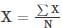Where, X = Mean, ∑X = sum of all items, N = number of items
Given, mean of 20 items is 55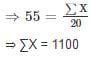⇒ two items are removed, so number of items = 18
⇒ removed items = 45 + 30 = 75
⇒ ∑X = 1100 – 75 = 1025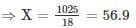Quantitative Aptitude - Test 6 - Question 21

The percentage of metals in a mine of lead ore is 60%. Now the percentage of silver is 3/4 % of metals and the rest is lead. If the mass of ore extracted from this mine is 8000 kg, then the mass (in kg) of lead is:

Detailed Solution for Quantitative Aptitude - Test 6 - Question 21

Mass of ore extracted from mine of lead ore = 8000 kg.
Percentage of metals in a mine of lead ore = 60%
Mass of metal = 60% of 8000
= (60/100) × 8000
= 4800kg.
Percentage of silver is 3/4 % of metals and the rest is lead.
Mass of silver = 3/4 % of 4800
= (3/400) × 4800
= 36kg.
Mass of lead = (4800 – 36)kg

= 4764 kg.

Quantitative Aptitude - Test 6 - Question 22

A sum becomes Rs, 10000 after 3 years and Rs, 20000 after 6 years on compound interest. The sum is

Detailed Solution for Quantitative Aptitude - Test 6 - Question 22

For CI: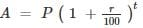Where,
A is the amount at the end of time t,
P is the principal,
t is time,
r is rate
A sum becomes Rs, 10000 after 3 years and Rs, 20000 after 6 years on compound interest.
For t = 3yrs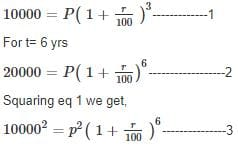Diving eq 3 by eq 2 we get,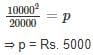Quantitative Aptitude - Test 6 - Question 23

The ratio of monthly incomes of A and B is 7: 8 and their monthly expenditures in ratio of 3: 4. If each of them saves Rs. 400 per month. Find the sum of their monthly income.

Detailed Solution for Quantitative Aptitude - Test 6 - Question 23

Given, ratio of monthly incomes of A and B is 7: 8.
Let the monthly incomes of A and B be 7a and 8a respectively, where a is any constant
Ratio of monthly expenditures is 3: 4
Let the monthly expenditures be 3b and 4b respectively, where b is any constant
Given, each of them saves Rs. 400
∴ 7a – 3b = 8a – 4b = 400
⇒ a = b
∴ 7a – 3a = 400
⇒ a = 100
Sum of their income = 7a + 8a = 15a
⇒ Sum of their income = Rs. 1500

Quantitative Aptitude - Test 6 - Question 24

The value of (1001)3 is

Detailed Solution for Quantitative Aptitude - Test 6 - Question 24

The value of (1001)= 1001 × 1001 × 1001
= 1003003001
Or,
1001 = 1000 + 1
Cubing
(1001)3 = (1000 + 1)3
We know that, (a + b)3 = a3 + b+ 3ab (a + b)
⇒ (1001)3 = 10003 + 1 + 3000 × (1000 + 1)
⇒ (1001)= 1000000000 + 1 + 3003000
⇒ (1001)= 1003003001

Quantitative Aptitude - Test 6 - Question 25

For any integral of n, 32n + 9n + 5 when divided by 3 will leave the remainder

Detailed Solution for Quantitative Aptitude - Test 6 - Question 25

32n + 9n + 5 = 3 × 32n-1 + 9n + 3 + 2
= 3(32n-1 + 3n + 1) + 2
As, 3(32n-1 + 3n + 1) is completely divisible by 3.
Therefore,
Remainder is 2

## SSC CGL Tier 1 Mock Test Series

42 docs|103 tests
 Use Code STAYHOME200 and get INR 200 additional OFF Use Coupon Code
Information about Quantitative Aptitude - Test 6 Page
In this test you can find the Exam questions for Quantitative Aptitude - Test 6 solved & explained in the simplest way possible. Besides giving Questions and answers for Quantitative Aptitude - Test 6, EduRev gives you an ample number of Online tests for practice

## SSC CGL Tier 1 Mock Test Series

42 docs|103 tests